# Matrix multiplication

If necessary, refer to the matrix notation page for a refresher on the notation used to describe the sizes and entries of matrices.

### Matrix-Scalar multiplication

The first kind of matrix multiplication is the multiplication of a matrix by a scalar, which will be referred to as matrix-scalar multiplication. A scalar is a number that makes things larger, smaller, or even negative (think of a negative scalar as "pointing backwards" or "flipping" something in the opposite direction). Given an m × n matrix, A, and a scalar, c, the matrix, cA, or Ac, is the m × n matrix whose i,jth entry is c times the i,jth entry of A. In other words,for all i=1,...,m and j = 1,...,n. for example,

### Properties of Matrix-Scalar multiplication

• Commutatitve: cA = Ac
• Associative: (cd)A = c(dA)
• Distributive over matrix addition: c(A + B) = cA + cB
• Distributive over scalar addition: (c + d)A = cA + dA

### Matrix-Matrix multiplication

To multiply two matrices, A and B, the number of columns of A must equal the number of rows of B. For a product matrix, AB, to be defined in general, A must be m × n, and B must be n × p, for some positive integers m, n, and p. If A is m × n, adn B is n × p, the product matrix, AB, is the m × p matrix such that the i,jth entry of AB, denoted (AB)i,j, is the dot product of the ith row vector of A

[ai,1, ..., ai,n]

with the jth column vector of B

[b1,j, ..., bn,j]T

Therefore, (AB)i,j is given by the formula:===for all i = 1,2 ..., m and j = 1,2, ..., p (i runs through the rows of A, of which there are m, and j runs through the columns of B, of which there are p). For example, if A is the following 2 × 3 matrix and B is the following 3 × 2 matrix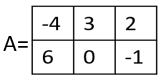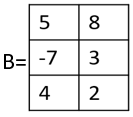then====corresponds to:===corresponds to:====corresponds to:

and====corresponds to:

Assembling these entries into the matrix product AB yields

 AB =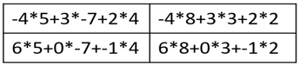=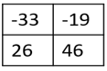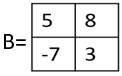then AB is not defined since A has 3 columns and B has 2 rows, and 3 ≠ 2.

### Properties of matrix multiplication

• Commutative with scalars (i.e. matrix-scalar multiplication above): If A is m × n, B is n × p, and c is a scalar, cAB = AcB = ABc. Note: matrix-matrix multiplication is not commutative. For example, matrix A × matrix B does not necessarily equal matrix B × matrix A and more typically does not.
• Associative: If A is m × n, B is n × p, C is p × q, then (AB)C = A(BC)